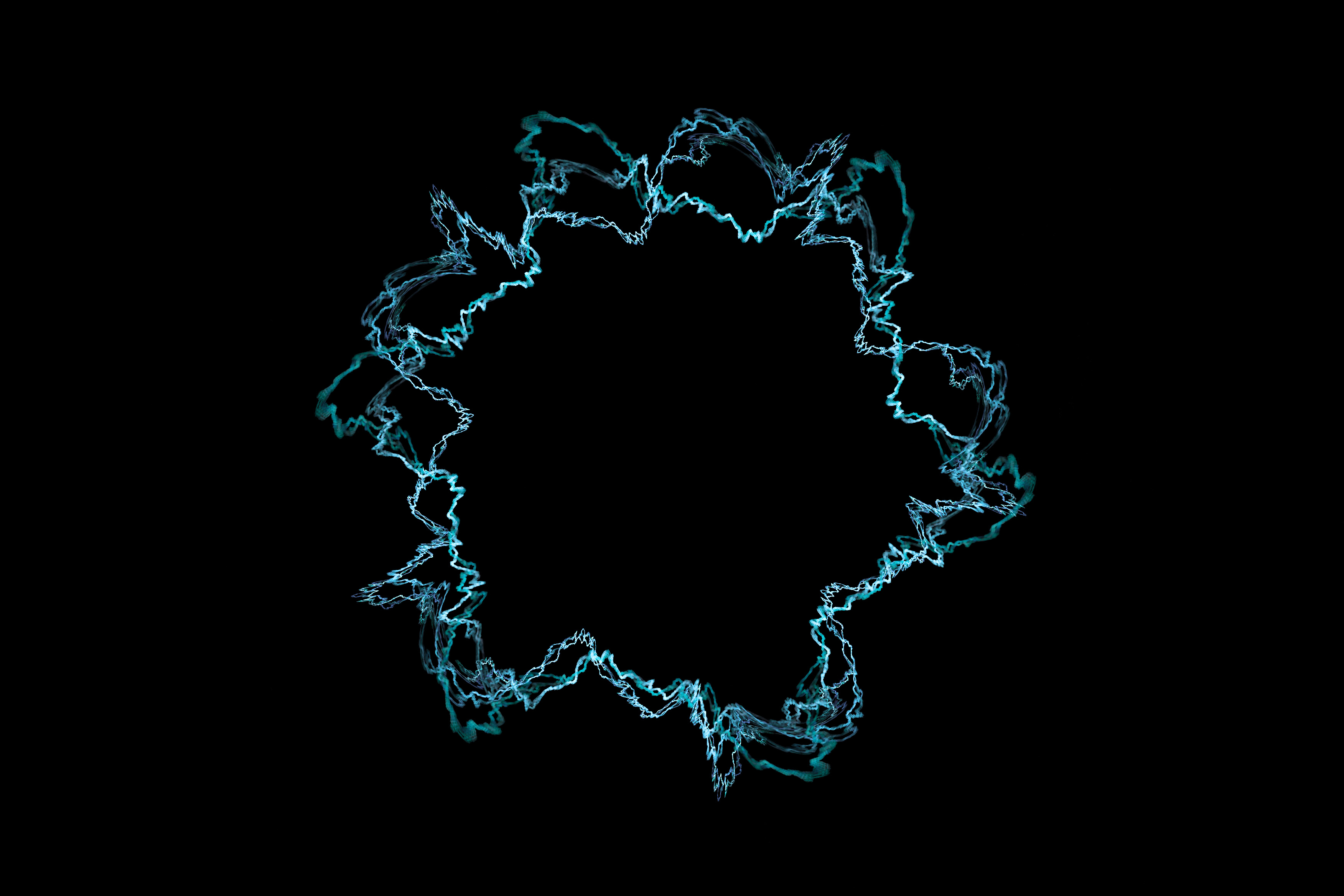# A string theorySuppose a piece of string is drawn taut between two endposts, and let $\phi(x,t)$ denote the height of the string above the line connecting the endposts at the point $x$ along the same line at time $t$. Which of the following differential equations correctly describes the evolution of the string shape over time?

Assumptions

• The composition of the string is uniform.
×